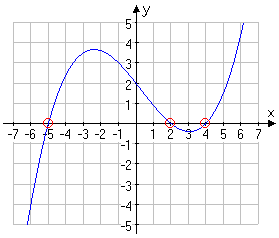x- and y-InterceptsThe graphical concept of x- and y-intercepts is pretty simple. The x-intercepts are where the graph crosses the x-axis, and the y-intercepts are where the graph crosses the y-axis. The problems start when we try to deal with intercepts algebraically.

To clarify the algebraic part, think again about the axes. When you were first introduced to the Cartesian plane, you were shown the regular number line from elementary school (the x-axis), and then shown how you could draw a perpendicular number line (the y-axis) through the zero point on the first number line. Take a closer look, and you'll see that the y-axis is also the line "x = 0". In the same way, the x-axis is also the line "y = 0".

Then, algebraically,

• an x-intercept is a point on the graph where y is zero, and
• a y-intercept is a point on the graph where x is zero.

More specifically,

• an x-intercept is a point in the equation where the y-value is zero, and
• a y-intercept is a point in the equation where the x-value is zero.

• Find the x- and y-intercepts of 25x2 + 4y2 = 9

Using the definitions of the intercepts, I will proceed as follows:

x-intercept(s):

y = 0 for the x-intercept(s), so:

25x2 + 4y2 = 9
25x2 + 4(0)2 = 9

25x2 + 0 = 9

x29/25

x = ± ( 3/5 )

Then the x-intercepts are the points ( 3/5, 0) and ( –3/5, 0)

x = 0 for the y-intercept(s), so:

25x2 + 4y2 = 9
25(0)2 + 4y2 = 9

0 + 4y2 = 9

y29/4

y = ± ( 3/2 )

Then the y-intercepts are the points (0, 3/2 ) and (0, –3/2 )

Just remember: Whichever intercept you're looking for, the other variable gets set to zero.

In addition to the above considerations, you should think of the following terms interchangeably:

"x-intercepts" = "roots" = "solutions" = "zeroes"

In other words, the following exercises are equivalent:

• Find the x-intercept(s) of y = x3 + 2x2x – 3
• Solve x3 + 2x2x - 3 = 0
• Find the zeroes / roots of  f(x) = x3 + 2x2x – 3

If you keep this equivalence in the back of your head, many exercises will make a lot more sense. For instance, if they give you something like the following graph:...and ask you to find the "solutions", you'll know that they mean "find the x-intercepts", and you'll be able to answer the question, even though they were clumsy in their use of the mathematical terms, and they never gave you the equation.